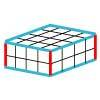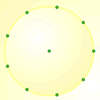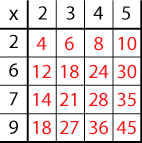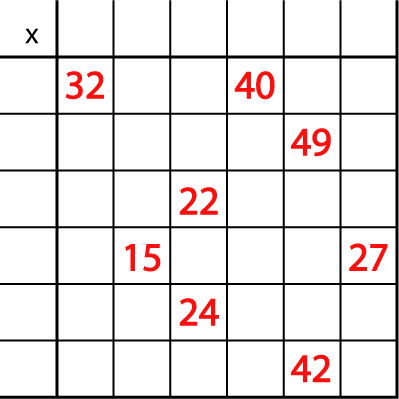#### You may also like### Round and Round the Circle

What happens if you join every second point on this circle? How about every third point? Try with different steps and see if you can predict what will happen.### Making Cuboids

Let's say you can only use two different lengths - 2 units and 4 units. Using just these 2 lengths as the edges how many different cuboids can you make?How many DIFFERENT quadrilaterals can be made by joining the dots on the 8-point circle?

# Mystery Matrix

##### Age 7 to 11Challenge Level

Have a look at this table square or matrix:Can you see how it has been constructed?
Why are some numbers in black and some in red?
Can you explain why the red 6 is in that particular square?
Why is there a 45 in the bottom right-hand corner?

You will notice that the numbers 2 to 9 are used to generate the matrix and only one of these numbers is used twice (the 2).

Can you fill in the matrix (table square) below?

The numbers 2 to 12 were used to generate it with, again, just one number used twice.You might like to have a go at similar versions of this task using the interactivity below.

Once you've worked out what each heading must be, drag the purple numbers to the appropriate spaces. When you think you have cracked it, click "Show the solution" to see if you are right.

For an extra challenge, you could try this 6 by 6 version of the grid.

You may like to try Missing Multipliers once you've had a go at this task.

You may be interested in the other problems in our What is the Question? Feature.